# Graphing Linear Functions

HIDE SOLUTIONS

1. Graph the function 3x + 2y = -4

Solution:

Step 1. Rewrite in the form y = a + bx and simplifyStep 2. Find a set of points which satisfy the equation and plot them.

let x = 0                       then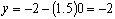let x = 2                       then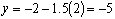let x = -2                      then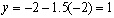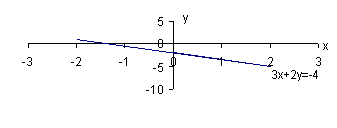2. Graph the function 2x –y = -1

Solution:

Step 1. Rewrite in the form y = a + bx and simplify

y = 1  + 2x

Step 2. Find a set of points which satisfy the equation and plot them.

let x = 0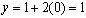let x = 2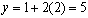let x = 1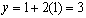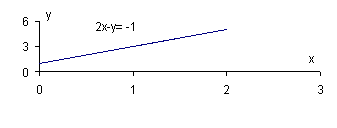3. A copy shop can produce a course reader at a cost of \$25 per copy. The monthly fixed costs are \$10,000.

a. Determine the total monthly cost as a function of the number of copies produced.

b. Graph the total monthly cost function.

Solution:

a. Total cost  =  fixed cost  + variable cost

C(q)    =  10,000  + 25 q

b. Find a set of points which satisfy the equation and plot them

 q C(q) 0 10,000 100 12,500 500 22,500 1,000 35,000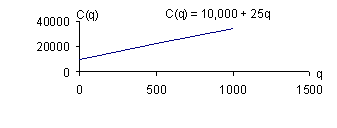4. A chemical plant was found to be discharging toxic waste into a waterway. The state in which the chemical plant was located fined the company \$125,000 plus \$1,000 per day for each day on which the company continued to violate water pollution regulation.

a. Express the total fine as a function of the number of days in which the company remains in non-compliance.

b. Graph the relationship.

Solution:

a. Let d = the number of days in which the company remains in non-compliance

f(d)  = 125,000  +   1000d

b. Find a set of points which satisfy the equation and plot them

 d f(d) 0 125,000 1 126,000 5 130,000 10 135,000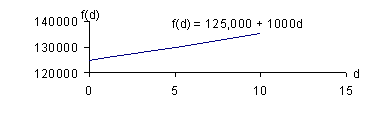[Index]